# Integration by substitution method

On the basis of the method is following simple feature of indefinite integral:We express initial integration variable x in terms of new variable t and get the expression for dx. Then we substitute derived expression into initial integral. We assume, that new variable is fitted such as new integral has the simplier form than initial.

## Example: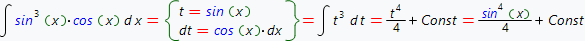we introduce new variable by using the formula: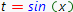then calculate the expression dt:after this, we substitute new expression into initial integral:When the change of variable is done, we get more simple integral: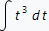which easily can be integrated: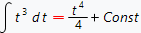after calculations is done, we should go back to the old variable: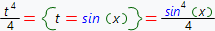thus, finally we get: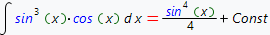Derived result can always be checked by the differentiation:## Next example: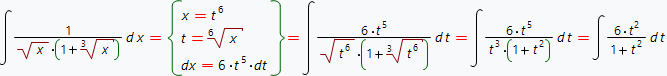continue: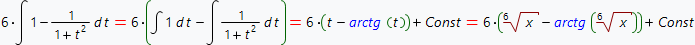Use our online integrals calculator which automatically determines and makes optimal variable substitution to calculate your integral with step by step solution.